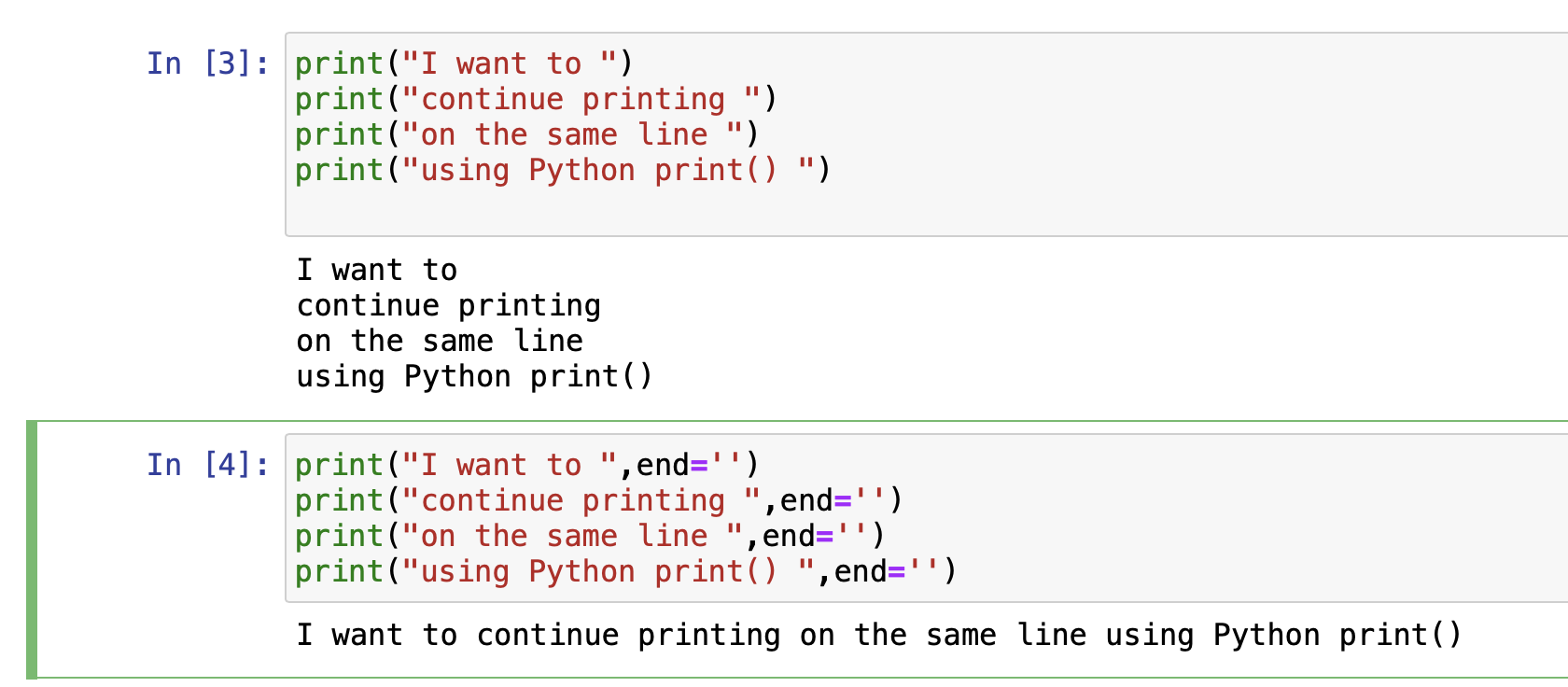# Python print() function without a newline using the end parameter

In Python, in order to print out text in the console, you can use the print() function. But by default, the print() will add a new line character (based on the OS \n, \r, or \r\n) at the end of the text that it printed out.

If you do not want to print text with a new line character at the end, you can use the end parameter of the print() function.

Example:
``````print("I want to ",end='')
print("continue printing ",end='')
print("on the same line ",end='')
print("using Python print() ",end='')``````
Output on the same line as expected:

I want to continue printing on the same line using Python print()## More on the "end parameter"

• The end parameter is used to specify the character or characters that should be printed at the end of the printed text using the print() function.

• By default end parameter is set to a newline character i.e. \n

• So when you use the default print() function in python, it always adds a newline character at the end of the text.

• If you wish to change it to any other character, you can simply use the parameter end= followed by a character.

Let's have some fun!

In the below example, we have replaced the end parameter with an emoji and a new line.

``````print("Hello ",end='😀\n')
print("How are you? ",end='😀\n')``````
Output:

Hello 😀
How are you? 😀

-

Have Questions? Post them here!